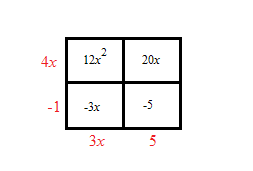### Home > CCA > Chapter 8 > Lesson 8.1.1 > Problem8-7

8-7.

Multiply the expressions below using a generic rectangle. Then verify Casey's pattern (that the product of one diagonal equals the product of the other diagonal). Homework Help ✎

1. $(4x−1)(3x+5)$12x² + 17x − 5

12x²(−5) = −3x(20x)
−60x² = −60x²

1. $(2x−7)^2$

(2x − 7)² = (2x − 7)(2x − 7)